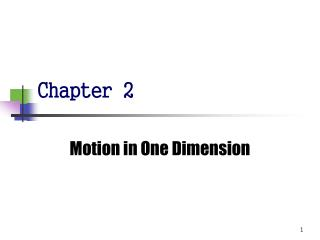DownloadDownload PresentationChapter 2

# Chapter 2

Télécharger la présentation## Chapter 2

- - - - - - - - - - - - - - - - - - - - - - - - - - - E N D - - - - - - - - - - - - - - - - - - - - - - - - - - -
##### Presentation Transcript

1. Chapter 2 Motion in One Dimension

2. 2.1 Kinematics • Describes motion while ignoring the agents that caused the motion • For now, will consider motion in one dimension • Along a straight line • Will use the particle model • A particle is a point-like object, has mass but infinitesimal size

3. Position 位置 • Defined in terms of a frame of reference • One dimensional, so generally the x- or y-axis • The object’s position is its location with respect to the frame of reference Fig 2.1(a)

4. Position-Time Graph • The position-time graph shows the motion of the particle (car) • The smooth curve is a guess as to what happened between the data points Fig 2.1(b)

5. Displacement 位移 • Defined as the change in position during some time interval • Represented as x • SI units are meters (m) x can be positive or negative • Different than distance – the length of a path followed by a particle

6. Average Velocity 平均速度 • The average velocity is rate at which the displacement occurs • The dimensions are length / time [L/T] • The SI units are m/s • Is also the slope of the line in the position – time graph

7. Displacement from a Velocity - Time Graph • The displacement of a particle during the time interval ti to tf is equal to the area under the curve between the initial and final points on a velocity-time curve Fig 2.1(c)

8. Active Figure 2.1 • If you can't see the image above, please install Shockwave Flash Player. • If this active figure can’t auto-play, please click right button, then click play. NEXT

9. 瞬時速度2.2 Instantaneous Velocity • The limit of the average velocity as the time interval becomes infinitesimally short, or as the time interval approaches zero • The instantaneous velocity indicates what is happening at every point of time

10. Fig 2.2(a)

11. Instantaneous Velocity, graph • The instantaneous velocity is the slope of the line tangent to the x vs. t curve • This would be the green line • The blue lines show that as t gets smaller, they approach the green line Fig 2.2(b)

12. Active Figure 2.2 • If you can't see the image above, please install Shockwave Flash Player. • If this active figure can’t auto-play, please click right button, then click play. NEXT

13. Instantaneous Velocity, equations • The general equation for instantaneous velocity is

14. Instantaneous Velocity, Signs • At point A, vx is positive • The slope is positive • At point B, vx is zero • The slope is zero • At point C, vx is negative • The slope is negative Fig 2.3

15. Instantaneous Speed • The instantaneous speed is the magnitude of the instantaneous velocity vector • Speed can never be negative • Thinking Physics 2.1(P.43)

16. Fig 2.4

17. 2.3 Constant Velocity • If the velocity of a particle is constant • Its instantaneous velocity at any instant is the same as the average velocity over a given time period • vx = vx,avg = Dx / Dt • Also, xf = xi + vxt • These equations can be applied to particles or objects that can be modeled as a particle moving under constant velocity

18. Fig 2.6

19. 2.4 Average Acceleration • Acceleration is the rate of change of the velocity • It is a measure of how rapidly the velocity is changing • Dimensions are L/T2 • SI units are m/s2

20. Instantaneous Acceleration • The instantaneous acceleration is the limit of the average acceleration as t approaches 0

21. Instantaneous Acceleration – Graph • The slope of the velocity vs. time graph is the acceleration • Positive values correspond to where velocity in the positive x direction is increasing • The acceleration is negative when the velocity is in the positive x direction and is decreasing Fig 2.7

22. QUICK QUIZ 2.3 Match each of the velocity-time graphs on the top with the acceleration-time graphs on the bottom. Fig 2.8

23. Active Figure 2.8 • If you can't see the image above, please install Shockwave Flash Player. • If this active figure can’t auto-play, please click right button, then click play. NEXT

24. Fig 2.9 The velocity of the car decrease from 30 m/s to 15 m/s in a time interval of 2.0 s a =?

25. Fig 2.10

26. 2.5 Negative Acceleration • Negative acceleration does not necessarily mean that an object is slowing down • If the velocity is negative and the acceleration is negative, the object is speeding up • Deceleration is commonly used to indicate an object is slowing down, but will not be used in this text

27. Acceleration and Velocity, 1 • When an object’s velocity and acceleration are in the same direction, the object is speeding up in that direction • When an object’s velocity and acceleration are in the opposite direction, the object is slowing down

28. Fig 2.11

29. 2.6 Kinematic Equations Fig 2.12

30. Active Figure 2.11 • If you can't see the image above, please install Shockwave Flash Player. • If this active figure can’t auto-play, please click right button, then click play. NEXT

31. Kinematic Equations • The kinematic equations may be used to solve any problem involving one-dimensional motion with a constant acceleration • You may need to use two of the equations to solve one problem • The equations are useful when you can model a situation as a particle under constant acceleration